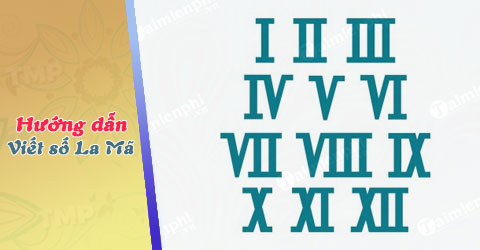Categories

# How to write and read roman numerals

Besides the Arabic numeral system 1, 2, 3 …, we also use a set of Roman numbers, but not everyone knows how to write and read Roman numbers correctly. In order to help readers better understand and master the writing and reading of these interesting numbers, we have compiled the content in the following article, inviting you to read.

Related posts

• How to write Roman numbers in Word 2019
• Solve homework on page 121 math textbook 3
• How to write Roman numbers in Word, index Roman numbers
• Postal code TP. How much is Ho Chi Minh?
• What is MD5?

Roman numerals (also known with other names – Roman numerals) are all the numbers born from ancient times, based on the Etruria, undergone modification and change in the Middle Ages. the perfect Roman numeral system is today. Each digit has a different value, let’s learn how to write and read this Roman number.How to write and read roman numerals

Learn about the origins of Roman numbers

Origin: Ancient Rome

Practical application: Instead of being used to calculate like ordinary natural numbers, Roman numerals are used to mark large items in a speech / text; write three chord notes in music; numbered for some major activity; write on dials to indicate hours and decorations; numbering the presidents …

### The best way to write Roman numbers

– There are 7 basic Roman numerals:

+ I = 1
+ V = 5
+ X = 10
+ L = 50
+ C = 100
+ D = 500
+ M = 1000

– In the Roman numeral system, there is no 0.
– Principles of writing a Roman number:

+ Adding to the right of the original digit means that we add the value for the original digit, but we cannot add more than 3 times.

For example: We can add the right number V = 5 as follows: VI = 6; VII = 7; VIII = 8; can not write VIIII.

+ Adding to the left of the original number means reducing the value for that digit and the digits can only be written once.

For example: – We can write I = 1 in front of V = 5 => IV = 4

– Cannot write: IIV

*Note :

+ The digits I, X, C, M must not repeat more than three times in a row
+ The digits V, L, D do not repeat more than once
+ One of the 7 basic digits is repeated 2 or 3 times showing their value 2 or 3 times. For example: I = 1; II = 2; III = 3

*For example : Write the following numbers as Roman numerals: 24, 1994, 2014, 2018

Instructions: How to write the numbers in turn as follows: XXIV; MCMXCIV; MMXIV; MMXVIII

### How to read Roman numbers for the standard?

Please follow our instructions on how to write and read Roman numerals: In order to correctly read a Roman numeral, you first need to master the above Roman numerical rules. When reading, it is necessary to divide the Roman number into thousands, hundreds, tens and units, and then read as ordinary natural numbers.

* Note :

– The number I = 1 only precedes the number V = 5 or X = 10, and does not appear before any other digit.
+ Correct writing: IV = 4; IX = 9
+ Can not write: IC, ID
– The digit X = 10 just comes before L = 50 or C = 100.
+ Correct writing: XL = 40; XC = 90
– The number C = 100 just comes before D = 500 or M = 1000.
+ Correct writing: CD = 400; CM = 900
+ Can not write: CX; CL

* For example : Read the following numbers: MMXX; MCMXC; MDXLVIII; CMLXXVIII

Instructions: Students read the numbers in turn as follows:

– MMXX = 2020: Two thousand and twenty.
– MCMXC = 1990: One thousand (thousand) nine hundred and ninety.
– MDXLVIII = 1548: One thousand (thousand) five hundred and forty-eight.
– CMLXXVIII = 978: Nine hundred and seventy eight.

Roman numerals are used only in basic arithmetic and hardly applied in calculation as natural numbers because Roman numerals are quite complex and complicated to write and read. However, if you have mastered the above basic principles, you will surely find that Roman numerals are very simple and interesting, right?

https://thuthuat.taimienphi.vn/cach-viet-va-doc-so-la-ma-35394n.aspx
So is writing Roman numbers in Word any different from the usual spelling? Please answer you is the way write Roman numbers in Word It’s also not difficult, you can refer to this article on ElectrodealPro offline.

.